Zhou, H., Ma, L., Sun, L., Zhou, H., Liu, D., and Cai, L. (2018). "Impact moderation of power transmission lines on the environment by the shielding of trees," BioRes. 13(4), 8239-8250.

#### Abstract

Dense high voltage power transmission lines limit human living space and affect the natural landscape and environment. Concerns about the environment in the corridor of high voltage power transmission lines and the occupied land area need to be addressed. Trees are a dissipative medium that can affect polarization in electric fields, leading to excitation of the induced electric field and shielding the impact of electric fields. A mathematical model for the electric field calculation of trees was established by selecting, mapping, and calculating the larch tree species in an experimental forest field at Northeast Forestry University, Heilongjiang Province, China. This study used the finite element method of equivalent excitation sources and the equivalent tree model to estimate the electric field shielding effectiveness of trees. The shielding effect of trees on the electric field of high voltage transmission lines was obvious. Planting trees reduced the transmission lines corridor width by 50.7%, and the maximum electric field in the ground decreased by 95.9%. Thus, planting trees to reduce the electric field of power transmission lines was more effective than the currently widely used method of erecting wires.

Impact Moderation of Power Transmission Lines on the Environment by the Shielding of Trees

Hongwei Zhou,a Ling Ma,a,* Liping Sun,a,* Hongjv Zhou,a Desheng Liu,b and Liping Cai c

Dense high voltage power transmission lines limit human living space and affect the natural landscape and environment. Concerns about the environment in the corridor of high voltage power transmission lines and the occupied land area need to be addressed. Trees are a dissipative medium that can affect polarization in electric fields, leading to excitation of the induced electric field and shielding the impact of electric fields. A mathematical model for the electric field calculation of trees was established by selecting, mapping, and calculating the larch tree species in an experimental forest field at Northeast Forestry University, Heilongjiang Province, China. This study used the finite element method of equivalent excitation sources and the equivalent tree model to estimate the electric field shielding effectiveness of trees. The shielding effect of trees on the electric field of high voltage transmission lines was obvious. Planting trees reduced the transmission lines corridor width by 50.7%, and the maximum electric field in the ground decreased by 95.9%. Thus, planting trees to reduce the electric field of power transmission lines was more effective than the currently widely used method of erecting wires.

Keywords: Trees; Transmission lines; Shielding; Electric field; Equivalent model; Finite element method

Contact information: a: Northeast Forestry University, Harbin, 150040, China; b: Jiamusi University, Jiamusi 154007, China; c: University of North Texas, Denton, TX 76201, USA;

* Corresponding authors: easyid@163.com, zdhslp@163.com

INTRODUCTION

In the construction of high voltage transmission lines, it is unavoidable to leave a corridor under the lines (Doukas et al. 2011; Gu et al. 2017). A high voltage transmission corridor is the designated space between two parallel lines, which are formed by extending a certain distance on both sides of the 35 kV and above ultra-high voltage (UHV) overhead power transmission lines, by calculating the maximum wind deflection and safe distance of the conductor (de Vasconcelos et al. 2017). The UHV transmission corridor needs a large amount of land because the UHV corridor width is over 100 meters. The demand of electricity expands as the economic development, but the enlargement of the UHV transmission corridor wastes land resources, which creates conflicts of interest (Luo et al. 2016). In China, the cost of land demolition of the UHV transmission line from Xiangjiaba to Shanghai is much higher than the cost of project construction (Yuasa et al. 2014).

A transient electric shock is an electric shock that occurs at the moment when a person is exposed to an electrostatic object in an electric field, and the charge accumulated on the induction object is released to the earth through the human body (Tenforde and Kaune 1987). Some research results indicated that the human body may feel a sting of electric shock if it touches a ground conductor when it was insulated with Earth at higher electric field intensity (Deadman et al. 1988). People in the high voltage transmission corridor are vulnerable to transient electric shocks, and there have been reports of transient electric shocks on residents near some UHV lines (Bracken 1976; Delaplace and Reilly 1978; Carstensen 1997). Although sometimes the discharge energy is small and presents no direct danger to people, it often creates a sting or panic (Subcommittee 1972). For this reason, Japan and the state of New Jersey (USA) have regulated the electric field intensity of the edge of UHV lines corridor at 3 kV/m (Kawada 2000; Zhao et al. 2013). To reduce the occurrence of transient electric shock, the electric field intensity near the ground must be controlled.

Ismail and Al-Kandari (2009) studied the electromagnetic environment near the 275-kV high voltage transmission lines in Kuwait. By planting a row of trees inside the transmission corridor to shield the electric field generated by UHV transmission lines, the boundary of the transmission corridor was reduced. A row of trees was equivalent to an infinite continuously long grounded cylindrical conductor. The charge simulation method demonstrated the effect of trees on reducing the span of transmission corridor and shielding the electromagnetic field. In a study of the electric field value near 500 kV transmission lines, Suojanen et al. (2000) found that the electric field measurement near the spruce trees was different from the calculated results. The spruce forests reduced the electric field near the transmission lines.

When solving the three-dimensional electric field around the trees under transmission lines, it is necessary to take into account the electromagnetic parameters of trees, the shape of trees, the gap between trees, etc., which are different from the calculation of two-dimensional electric field (Costache 1987; Khebir et al. 1990a,b; Lu et al. 2007). However, in the electric field of transmission lines calculation model, the thin conductor, the large air area and the huge number of grid subdivision can be used. To make the effective calculation based on the principle of equivalence, this study established an equivalent model of transmission lines, and tree matching the physical dimension and the solution area, which can effectively reduce the subdivision grid. Using this method, when the tree was adjacent to the transmission line, the shielding situation of electric field was calculated. The study concludes that planting trees could weaken the influence of electric field of transmission lines on the environment to address the challenge of increasing demand of large land area for transmission corridor by reducing transient electric shock.

EXPERIMENTAL

Modeling

Innovation model of transmission lines

The finite element method was used to calculate the distribution of the electric field around the transmission lines and the effect of the trees in the electric field. A three-dimensional model was used for the analysis of transmission lines. The diameter of the wires was 30 mm, the wire sag was 12 m, and the span was 400 m. The three-dimensional model established in Maxwell modeling and simulation software (http://www.aonesoft.net/ansys/maxwell.html) creates a huge volume difference between the slender conductor and the earth and the surrounding space, which led to a longer calculation-time for the subdivision grid and higher requirements for the configuration of the computer. Therefore, an equivalent model that solves the problem of a huge dimensional difference between two objects is needed.

The spatial potential distribution of actual transmission lines was calculated in Maxwell software to reduce the number and complexity of meshes subdivision, reducing computer storage, and speeding up calculation. According to the equivalent principle, the model of 8-split conductor of actual transmission lines is equivalent to per phase single conductor (Ma et al. 2014). Because the change of electric field near the conductor did not affect the electric field around the trees, instead of actual transmission lines, the equivalent model was utilized separately by the mathematical calculation and finite element simulation to verify the feasibility of the equivalent model as follows. The application steps of the equivalent model of transmission lines are listed below.

This study adopted the Ximeng-Shandong 1000 kV UHV AC transmission line, which started operation in August 2016. The type of tower used in the line was the cup-tower with a line rated voltage of 1000 kV, a conductor type of LGJ-500/45, an 8-split bundle conductor, a shielding wire type of JLB20A-240, and a shielding wire surface potential of 0. The line voltage adopted a maximum operating voltage of 1050 kV, a maximum arc sag of 12 m, and the lowest distance from the ground of 27 m. The coordinate origin was located at the central span (400 m span). The conductor distribution is shown in Fig. 1.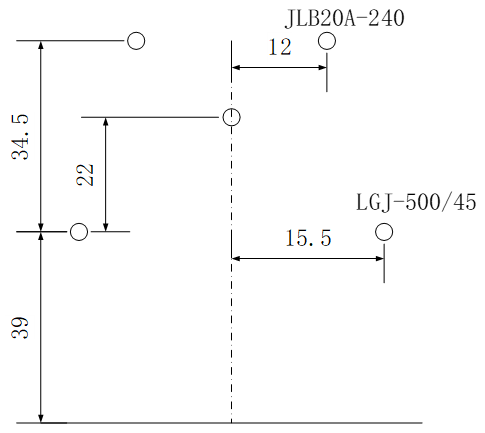Fig. 1. Conductor distribution diagram of the cup-tower type transmission line

Because the voltage of every phase line changed, the Maxwell software was used to calculate the static electric field at a certain moment around three-phase AC transmission lines. Therefore, to calculate the maximum occupied area of the transmission corridor, the right-side conductor line voltage was considered as the maximum value, and the line voltages of the other two conductors were calculated. The maximum value of the width of the single side transmission corridor was obtained, and the width of the total transmission corridor can be derived by symmetry, according to Eqs. 1, 2, and 3,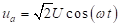(1)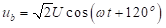(2)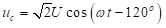(3)

where U is the phase voltage and t is the time.

Using Eqs. 1, 2, and 3, it was found that when the line voltage at the right conductor was 1050 kV, and the other two-phase line voltages were -525 kV.

The cross section of the maximum arc sag of the transmission line was used as the two-dimensional transmission line model. The model was established in Maxwell and the potential distribution of the surrounding space was calculated. Through the potential distribution of the transmission line in the two-dimensional space, as shown in Fig. 2a, the potential iso-surfaces of 500 kV, -250 kV, and -250 kV were respectively utilized to establish a single equivalent conductor instead of the original eight-split conductor. The original model was applied, and the conductor excitation was 500 kV, -250 kV, or -250 kV. A two-dimensional equivalent transmission line model was reconstructed with the plotted equipotential surface curve, as shown in Fig. 2b.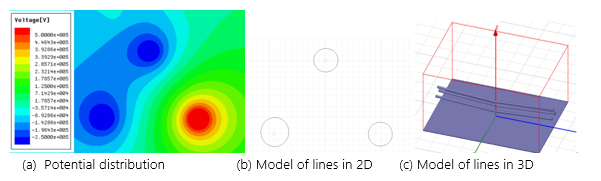Fig. 2. Models of transmission lines

A two-dimensional equivalent model of transmission line was used as the cross section located at the maximum arc sag of the three-dimensional equivalent model of the transmission line. According to the catenary of the conductor as a path, a three-dimensional equivalent transmission lines model was obtained along the path scanning, as shown in Fig. 2c.

Equivalent model of trees

For the three-dimensional finite element calculation, the tree was divided into three parts to establish the models: trunks, branches, and leaves. Because the number of mesh subdivisions of the finite elements was too large to be calculated, it was proposed that the tree shape was equivalent to the regular shape, in which, tree was divided into two parts: the trunk and the crown, to give the equivalent electromagnetic parameters. The crown was mainly composed of branches, leaves, and air. According to the solution theory of electromagnetic field inverse problem, the electromagnetic parameters equivalent to the crown can be deduced from the influence of a tree on an electric field.

Application steps of tree equivalent model

(1) Three-dimensional tree shape modeling

For the three-dimensional trees in summer and winter model, the further equivalent simplified treatment was needed with the long axis as the crown length, and the short axis as the crown diameter. The crown was equivalent to ellipsoid.

Determination of the electromagnetic parameters of trees

Based on the established three-dimensional equivalent transmission lines model, the established tree model was placed on the ground below the cross section of the maximum arc sag of the transmission line. The permittivity of the crown model was 5000, the conductivity was 0.001 S/m. The permittivity of the trunk model was 5000, and the conductivity was 0.1 S/m. The tree model was moved along the x-axis until the maximum electric field over the ground 1.5 m was equal to 4 kV/m. At this time, the trees were located at 24.8 m and the maximum electric field position was 37.4 m.

A three-dimensional equivalent transmission lines model was re-established. The equivalent tree model was set up at 24.8 m. The actual crown of a tree was composed of branches, leaves, air, and other substances, and the equivalent model only simplifies the crown further. After the equivalent simplification, this study considered it as an ellipsoid of homogeneous medium. Therefore, the trunks’ electromagnetic parameters in the equivalent model were the same as those in the original model. The crown’s electromagnetic parameters in the tree model were changed, until the maximum electric field was 4 kV/m over the ground and 1.5 m behind the tree. The electromagnetic parameters of the equivalent model can be obtained by utilizing the inverse calculation of finite element method, as shown in Table 1.

Table 1. Electromagnetic Parameters of Equivalent Model and Tree Model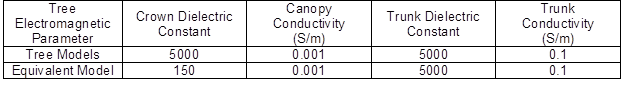Accuracy verification of tree equivalent model

Generally, substances can be sorted by composition, as single media or mixed media. For example, pure water belongs to a single medium while a living body is a mixed media (Dobson et al. 1985). If the dielectric constant and the volume ratio of each component are known in the mixed medium, the effective dielectric constant of the mixture can be calculated using the Debye-Cole equation, as shown in Eq. 4 (Chuah et al. 1997),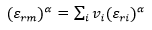(4)

where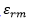is the effective dielectric constant of the mixture,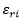is the dielectric constant of the i component,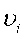is the volume ratio of the i component, and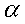is the empirical constant. The sum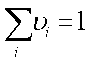;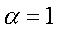indicates that the dielectric constant of mixed medium is linearly related to the volume ratio of each component.

In the equivalent model, the crown was regarded as an ellipsoid with a homogeneous medium, and the crown contained branches, leaves, air, and other components that also belonged to the mixed media. Because the crown of the winter tree model was simplified as an ellipsoid, it was necessary to calculate the dielectric constant of the equivalent tree crown. The tree crown of the winter tree only contained branches and air, and was calculated using Eq. 5,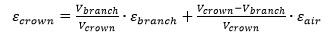(5)

where Vbranch is the volume of the branches, Vcrown is the volume of the crown,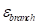is the dielectric constant of the branches,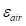is the dielectric constant of air.

Using the modeling program SolidWorks, the total volume of branch parts in the winter model was calculated,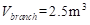. The volume of an ellipsoid was found using Eq. 6,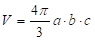(6)

where a, b, and c represent the radial length of the x, y, and z axes.

The values of ab, and c where found to be 2.8 m, 2.8 m, and 2.56 m, respectively. Therefore,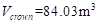,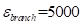, and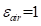. The dielectric constant of the equivalent crown was then calculated as,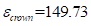.

It is feasible to solve the electric field around the transmission lines by the finite element method, and to deduce the equivalent dielectric constant of trees according to the theory of electromagnetic inverse.

RESULTS AND DISCUSSION

Calculation on the Effect of Trees

The distribution of the electric field was influenced by all kinds of objects under the transmission lines. The complex environment, plants, and cement concrete belong to the dielectric; while the building’s reinforcement, doors, and windows belong to the conductor (Arabi et al. 1991; J-Fatokun et al. 2010). In actual transmission lines, the most common objects are vegetation and buildings (Wan et al. 2010). In this study, the influence of trees on the electric field of transmission lines was investigated. According to the reference, the control value of electric field intensity at the edge of the transmission corridor was 4 kV/m. The width of the transmission corridor was 49.3 m at the right side, which was found through the calculation of the equivalent transmission lines above. To examine the shielding and distortion effects of trees on electric fields, and to reduce the width of the transmission corridor, a row of tree models was planted at 49.3 m parallel to the transmission lines. The larch tree was used to develop equivalent model of larch.

The information of the tree included: a tree height of 11.81 m, a crown length of 5.11 m, a branch height of 6.7, a crown diameter of 2.8 m, and breast height diameter of 0.1446 m.

The tree model was divided into two parts: the crown and the trunk. The crown dielectric constant and the conductivity were found to be 125 and 0.001 S/m, respectively. The dielectric constant and conductivity of the trunk was found to be 5000 and 0.1 S/m, respectively. Due to the shielding effect of trees on electric fields, the tree model was moved parallel to the transmission lines. The electric field had a value much smaller than 4 kV/m when located behind the trees, which was measured when the trees were not moving. As the trees moved towards the origin, the overall electric field behind the trees gradually increased. The shielding effect of trees on the electric field reached a maximum when the maximum value of the electric field at any point behind the tree reached 4 kV/m. At this point, the center of the tree was 24.8 m from the center of the transmission lines.

As can be seen in Fig. 3, the presence of trees had a positive shielding effect on the power frequency electric fields. At 24.8 m, the electric field decreased to nearly 0 V/m, with a decay of 95.9%. In the rear of the tree, a greater distance resulted in a rapid increase in the electric field. The electric field reached a maximum value of 4 kV/m at 37.4 m. As the distance increased beyond 37.4 m, the electric field decreased gradually, and the two curves became consistent after 100 m. The comparison showed that trees had a shielding effect on the electric field that greatly reduced the electric field behind the trees. After the tree shielding, the semi-width of the transmission corridor decreased from 49.8 m to 24.8 m, the width of transmission corridor decreased by 50 m in total, and the transmission corridor decreased by 50.7%.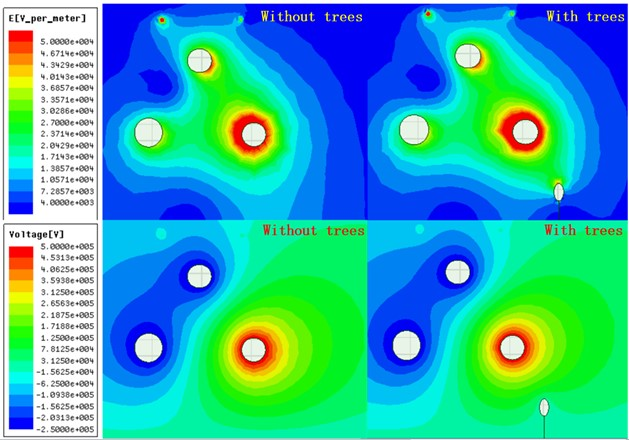(a) Influence of trees on the electric field and potential distribution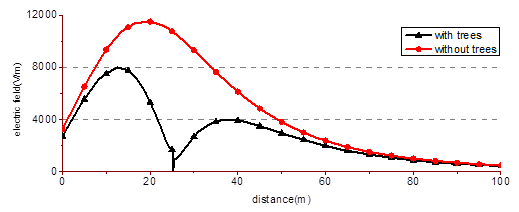(b) Contrast diagram of electric field intensity from 1.5 m to ground

Fig. 3. Contrast diagrams of electric field influence (a) and intensity (b) of transmission lines with and without trees

Comparison between Trees and Shielding Wires

Liu et al. (2011) developed a method to reduce the effect of transmission lines on people living in residential areas. This method involved erecting shielding wires below the transmission lines to weaken the power frequency of the electric field generated by the transmission lines.

Model of shielding wires

In the previous report, Liu et al. (2011) used a 220-kV transmission line from tower #54 to tower #55, with both tower types being cat head. A conductor had a cross-sectional area of 240 mm2, and the double split and split spacing of 40 cm. The house was located between tower #54 and tower #55, and at 109 m from tower #55. The distance of the third-floor balcony from the nearest side was 5.2 m, the lowest point of the third-floor balcony was 10.3 m, and the net height of the balcony was 1 m. The nearest three points were labeled A, B, and C, in which the conductor was closest to the balcony. The height of points A, B, and C were 20.7 m, 25.5 m, and 20.5 m, respectively. The distance between points A and B, and points B and C, was 6.4 m and 6.7 m, respectively. The exact location is shown in Fig. 4, and the positions of Land Lb are shown as horizontal and vertical observation lines, respectively.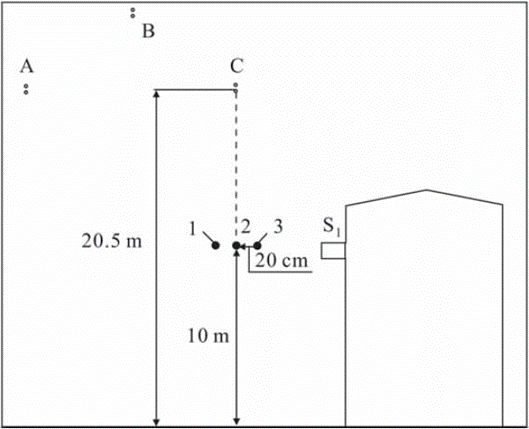Fig. 4. Position of shielding wires in literature (Liu et al. 2011)

As shown in Fig. 4, underneath the C-phase conductor, three shielding wires were erected 10 m above the ground at points 1, 2, and 3. The diameter of each of the shielding wires was 7 mm, and the interval between them was 20 cm.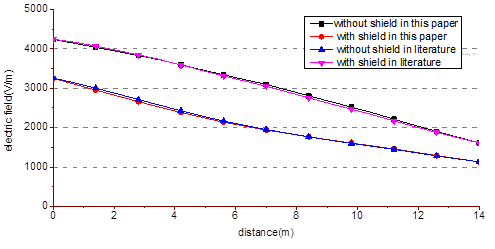Fig. 5. Comparison of the results between this method and that of Liu et al. (2011)

Simulation results of electric field with shielding wires

Transmission lines with and without shielding wires were simulated in this study. The electric field of the observation line La was calculated and compared to the electric field at 1.5 m above ground in different conditions without shielding wires and with three shielding wires. The results are shown in Fig. 5. As can be seen in the figure, the results of this study were consistent with those in the literature (Liu et al. 2011), showing that the proposed methods in this paper are reliable and have good accuracy.

The model of transmission lines with trees

The shielding effect of trees and shielding wires on of the electric field were compared. The parameters of the transmission lines are listed in Fig. 1. The trees were located 8.5 m from the center of the transmission line, the position of the lines and trees is shown in Fig. 6.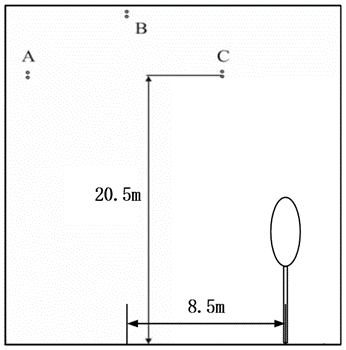Fig. 6. The position of lines and trees

Comparison of Shielding Effect between Trees and Shielding Wires

The method of this study was used to calculate the model in Fig. 6, and the shielding results of electric field by trees are shown in Fig. 7 and Table 2.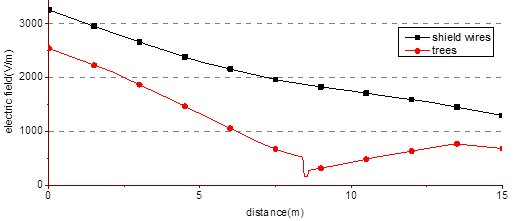Fig. 7. Comparison between the shielding effects of shielding wires and trees

Table 2. Electric Field Comparison between Shielding Wires and Trees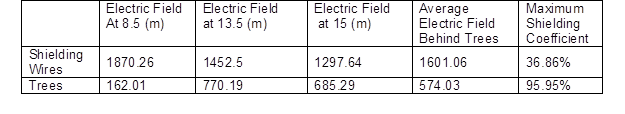When trees were planted at 8.5 m, the maximum electric field appeared at 13.5 m, with an electric field value of ETmax = 770.19 V/m. The electric field value with shielding wires was EL= 1452.5 V/m at this point, and the shielding effect of the shielding wires on the electric field was only 53.03% of that of the tree. After comparing the effect of trees and shielding wires on the electric field, the shielding effect of the planted trees on the electric field was much more effective than that of the shielding wires.

CONCLUSIONS

1. The equivalent excitation source model and the equivalent tree model were proposed to calculate the electric field of trees near power transmission lines. The electric field distribution in the surrounding space of the equivalent models and the original models were compared, and the potential distribution of the equivalent models and the original models were the same below the conductor and over the ground. The equivalent models can be used instead of the original transmission line model for simulation.
2. The dielectric constants of the equivalent tree model were deduced based on the simulation results of the tree model. Through the comparison of the theoretical calculation, it was concluded that the equivalent tree model can be applied to the electric field simulation of the trees near the power transmission line.
3. The equivalent model of trees near the transmission line was simulated by the finite element method. Trees had an obvious shielding effect on the power frequency electric field at 1.5 m over the ground. After comparing these results to the transmission line model using the method of shielding wires, the shielding effect of the electric field of trees was much greater than that of the shielding wires.

ACKNOWLEDGMENTS

The authors are grateful for the support of the National Key Research and Development Program (2017YFD0600101), the Central University Basic Research and Operating Expenses of Special Funding (2572016CB04) and the Harbin Application Technology Research and Development Projects (2016RQQXJ134).

REFERENCES CITED

Arabi, T. R., Djordjevic, A. R., Harrington, R. F., Murphy, A. T., and Sarkar, T. K. (1991). “On the modeling of conductor and substrate losses in multiconductor, multidielectric transmission line systems,” IEEE Transactions on Microwave Theory and Techniques 39(7), 1090-1097. DOI: 10.1109/22.85374

Bracken, T. D. (1976). “Field measurements and calculations of electrostatic effects of overhead transmission lines,” IEEE Transactions on Power Apparatus and Systems 95(2), 494-504. DOI: 10.1109/T-PAS.1976.32129

Carstensen, E. L. (1997). “Biological effects of power frequency electric fields,” Journal of Electrostatics 39(3), 157-174. DOI: 10.1016/S0304-3886(97)00003-X

Chuah, H. T., Chye, Y. H., and Kam, S. W. (1997). “Microwave dielectric properties of rubber and oil palm leaf samples: Measurement and modelling,” International Journal of Remote Sensing 18(12), 2623-2639. DOI: 10.1080/014311697217503

Costache, G. (1987). “Finite element method applied to skin-effect problems in strip transmission lines,” IEEE Transactions on Microwave Theory and Techniques 35(11), 1009-1013. DOI: 10.1109/TMTT.1987.1133799

de Vasconcelos, J. A., Teixeira, D. A., and de Oliverira Ribeiro, M. F. (2017). “Optimal selection and arrangement of cables for compact overhead transmission lines of 138/230 kV,” IEEE Latin America Transactions 15(8), 1460-1466. DOI: 10.1109/TLA.2017.7994793

Deadman, J. E., Camus, M., Armstrong, B. G., Héroux, P., Plante, M., and Thériault, G. (1988). “Occupational and residential 60 Hz electromagnetic fields and high-frequency electric transients: Exposure assessment using a new dosimeter,” The American Industrial Hygiene Association Journal 49(8), 409-419. DOI: 10.1080/15298668891379981

Delaplace, L. R., and Reilly, J. P. (1978). “Electric and magnetic field coupling from high voltage AC power transmission lines–Classification of short-term effects on people,” IEEE Transactions on Power Apparatus and Systems 97(6), 2243-2252. DOI: 10.1109/TPAS.1978.354728

Dobson, M. C., Ulaby, F. T., Hallikainen, M. T., and El-rayes, M. A. (1985). “Microwave dielectric behavior of wet soil-Part II: Dielectric mixing models,” IEEE Transactions on Geoscience and Remote Sensing 23(1), 35-46. DOI: 10.1109/TGRS.1985.289498

Doukas, H., Karakosta, C., Flamos, A., and Psarras, J. (2011). “Electric power transmission: An overview of associated burdens,” International Journal of Energy Research 35(11), 979-988. DOI: 10.1002/er.1745

General Systems Subcommittee (1972). “Electrostatic effects of overhead transmission lines Part I-Hazards and effects,” IEEE Transactions on Power Apparatus and Systems 91(2), 422-426. DOI: 10.1109/TPAS.1972.293224

Gu, S., Wan, S., Wang, J., Chen, J., and Li, T. (2017). “Development and application of ± 500 kV DC transmission line arrester in China power grid,” IEEE Transactions on Power Delivery 33(1), 209-217. DOI: 10.1109/TPWRD.2017.2652479

Ismail, H. M., and Al-Kandari, A. M. (2009). “Impact of using natural trees on the electric field reduction of Kuwait high voltage transmission systems,” Kuwait J. Sci. Eng 36(1B), 131-145. http://www.pubcouncil.kuniv.edu.kw/KJS/files/05Oct2013103036Impact.pdf

J-Fatokun, F., Jayaratne, R., Morawska, L., Birtwhistle, D., Rachman, R., and Mengersen, K. (2010). “Corona ions from overhead transmission voltage powerlines: Effect on direct current electric field and ambient particle concentration levels,” Environmental Science & Technology 44(1), 526-531. DOI: 10.1021/es9024063

Kawada, M. (2000). “Effect of ELF electric and magnetic fields exposure in consideration of the Japanese technology standard of the electric installation on the EEG spectra of human,” IEEJ Transactions on Power and Energy 120(8-9), 1127-1135. DOI: 10.1541/ieejpes1990.120.8-9_1127

Khebir, A., Kouki, A. B., and Mittra, R. (1990a). “Asymptotic boundary conditions for finite element analysis of three-dimensional transmission line discontinuities,” IEEE Transactions on Microwave Theory and Techniques 38(10), 1427-1432. DOI:10.1109/22.58681

Khebir, A., Kouki, A. B., and Mittra, R. (1990b). “Higher order asymptotic boundary condition for the finite element modeling of two-dimensional transmission line structures,” IEEE Transactions on Microwave Theory and Techniques 38(10), 1433-1438. DOI: 10.1109/22.58682

Liu, G., Xu, B., Ji, Y. F., Gao, Y. P., Xie, X. Z., and Zeng, N. X. (2011). “Control of power frequency electric field near building close to 220 kV transmission line,” High Voltage Engineering 37(12), 2904-2910. DOI: 10.13336/j.1003-6520.hve.2011.12.011

Lu, T., Feng, H,, Zhao, Z., and Cui, X. (2007). “Analysis of the electric field and ion current density under ultra high-voltage direct-current transmission lines based on Finite Element Method,” IEEE Transactions on Magnetics 43(4), 1221-1224. DOI: 10.1109/TMAG.2006.890960

Luo, B., Shi, J., Hu, S., and Zhuo, R. (2016). “New research progress of HVDC transmission technology in China Southern Power Grid Corporation,” in: IEEE International Conference on High Voltage Engineering and Application, Chengdu, China, pp. 1-5. DOI: 10.1109/ICHVE.2016.7800921

Ma, A., Xu, D., Zhang, Z., Li, F., and Zhao, L. (2014). “The nominal field strength calculation of UHVDC transmission lines based on surface charge method,” International Conference on Power System Technology IEEE, Chengdu, China, pp. 2242-2246. DOI: 10.1109/POWERCON.2014.6993738

Suojanen, M., Vehmaskoski, J., Kuusiluoma, S., Trygg, P., and Korpinen, L. (2000). “Effect of spruce forest on electric fields caused by 400 kV transmission lines,” in: PowerCon 2000 International Conference on Power System Technology, Perth, Australia, Vol. 3, pp. 1401-1405. DOI: 10.1109/ICPST.2000.898174

Tenforde, T. S., and Kaune, W. T. (1987). “Interaction of extremely low frequency electric and magnetic fields with humans,” Health Physics 53(6), 585-606. DOI: 10.1097/00004032-198712000-00002

Yuasa, H., Imagawa, T., Nakanishi, M., Niihara, S., Higashi, M., and Chen, Y. (2014). “The application of fault location system for 220kV overhead power line in China,” in: 9th IET International Conferenceon Advances in Power System Control, Operation and Management, Hong Kong, China, pp. 1-6. DOI: 10.1049/cp.2012.2128

Zhao, Z., Dong, S., Deng, X., and Jin, X. (2013). “Safety distance of UHV double-circuit AC transmission lines on one tower adjacent to buildings,” High Voltage Engineering 39(12), 2943-2950. DOI: 10.3969/j.issn.1003-6520.2013.12.015

Article submitted: June 19, 2018; Peer review completed: August 18, 2018; Revised version received and accepted: September 11, 2108; Published: September 18, 2018.

DOI: 10.15376/biores.13.4.8239-8250